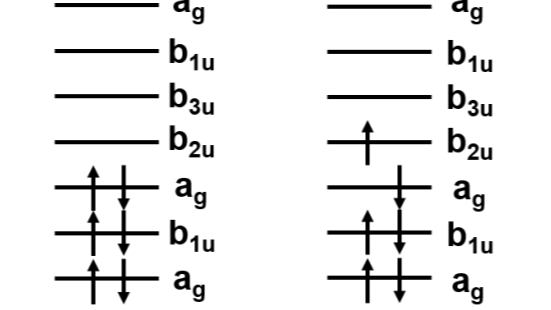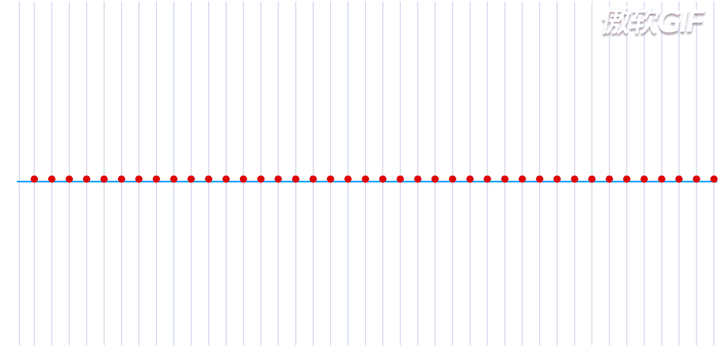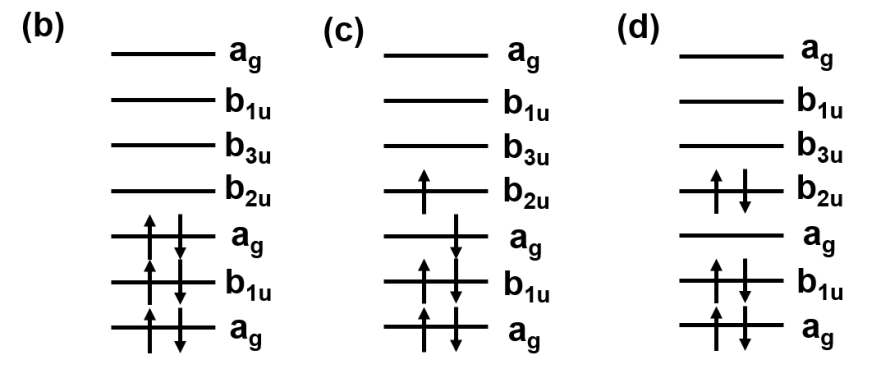# 二次量子化与量子计算化学量子计算机，是由基本单元量子比特所组成的新型计算体系，通过量子叠加和量子纠缠的特性，来完成对量子态的操纵，最终再通过量子测量获得到想要的计算结果。而在量子计算机上面执行量子化学的任务，被认为是一个非常promising的应用场景，不论是从最初费曼的想法与设计，还是这几年所发展起来的近期量子计算（NISQ）的技术，都对量子计算化学这一新兴研究方向进行了阐述。本文通过最基础的谐振波，讲解到薛定谔方程和动量算符的由来，最终介绍了两种量子化的变换。其实所谓的量子化，都是对表征体系进行了调整。一次量子化将哈密顿量从电子的粒子性带到了量子力学中的波粒二象性，引入了动量算符。二次量子化将动量表象和位置表象变换到粒子数表象，通过统计平均的方法去研究电子在不同轨道之间跃迁时的能量吸收与产生，用于表示体系总能量。

# 一维无限长谐振子模型$y(t)=Acos(\omega t+\phi_0)$

$y(x,t)=Acos(kx-\omega t+\phi_0)$$\left|\psi\right>=\psi(x,t)=Ae^{i(kx-\omega t+\phi_0)}=Acos(kx-\omega t+\phi_0)+iAsin(kx-\omega t+\phi_0)$

# 薛定谔方程的启发式推导

$\frac{\partial \psi}{\partial t}=-i\omega\psi,\frac{\partial \psi}{\partial x}=ik\psi,\frac{\partial^2\psi}{\partial x^2}=-k^2\psi$

$i\hbar\frac{\partial\psi}{\partial t}=\hbar\omega\psi=E_{wave}\psi$

$p\psi=\hbar k\psi=-i\hbar\frac{\partial}{\partial x}\psi\\ p^2\psi=\hbar^2 k^2\psi=-\hbar^2\frac{\partial^2}{\partial x^2}\psi\\ i\hbar\frac{\partial}{\partial t}\psi=-\frac{\hbar^2}{2m}\nabla^2\psi+U(x)\psi$

# 量子力学算符

$\hat{p}=-i\hbar\frac{\partial}{\partial x}$

\begin{align*} [x,p]f(x)&=x\hat{p}f(x)-\hat{p}xf(x)\\ &=-i\hbar\frac{\partial f(x)}{\partial x}+i\hbar\frac{\partial(xf(x))}{\partial x}\\ &=-i\hbar\frac{\partial f(x)}{\partial x}+i\hbar f(x)+i\hbar\frac{\partial f(x)}{\partial x}\\ &=i\hbar f(x)\Rightarrow[x,p]=i\hbar \end{align*}

$H=\frac{p^2}{2m}+U(x)\Rightarrow\hat{H}=-\frac{\hbar^2}{2m}\nabla^2+U(x)=i\hbar\frac{\partial}{\partial t}$

$\frac{\partial H}{\partial p}=\frac{p}{m}=\dot{x},\frac{\partial H}{\partial x}=\frac{\partial U(x)}{\partial x}=-F=-m\ddot{x}=-\dot{p}$

# 通用电子结构问题与BO近似

$H_{total}=\sum_{i=1}^K\frac{p_i^2}{2M_i}+\sum_{i=1}^N\frac{p_i^2}{2m_e}-\sum_{i,j}\frac{e^2}{4\pi\epsilon_0}\frac{Z_j}{\left|r_i-R_j\right|}+\frac{1}{2}\sum_{i\neq j}\frac{e^2}{4\pi\epsilon_0}\frac{1}{\left|r_i-r_j\right|}+\frac{1}{2}\sum_{i\neq j}\frac{e^2}{4\pi\epsilon_0}\frac{Z_iZ_j}{\left|r_i-r_j\right|}$

$\psi(r,R)=\chi(R)\psi(R;r)$

$H(p,r)=\sum_{i=1}^N\frac{p_i^2}{2m_e}-\sum_{i,j}\frac{e^2}{4\pi\epsilon_0}\frac{Z_j}{\left|r_i-R_j\right|}+\frac{1}{2}\sum_{i\neq j}\frac{e^2}{4\pi\epsilon_0}\frac{1}{\left|r_i-r_j\right|}$

# 一次量子化

$H=-\sum_i\frac{\nabla^2_i}{2}-\sum_{i,j}\frac{Z_j}{\left|r_i-R_j\right|}+\frac{1}{2}\sum_{i\neq j}\frac{1}{\left|r_i-r_j\right|}$

$\psi(\bold{x_0},…,\bold{x_{N-1}})= \frac{1}{\sqrt{N!}}\left|\begin{matrix} \phi_0(\bold{x_0})&\phi_1(\bold{x_0})&…&\psi_{M-1}(\bold{x_0})\\ \phi_0(\bold{x_1})&\phi_1(\bold{x_1})&…&\psi_{M-1}(\bold{x_1})\\ .&.&.&.\\ .&.&.&.\\ .&.&.&.\\ \phi_0(\bold{x_{N-1}})&\phi_1(\bold{x_{N-1}})&…&\psi_{M-1}(\bold{x_{N-1}}) \end{matrix} \right|$

$\psi(\bold{x_0},\bold{x_1},…,\bold{x_{N-1}})=-\psi(\bold{x_1},\bold{x_0},…,\bold{x_{N-1}})$

# 二次量子化

$H=\sum_{p,q}h_{pq}a^{\dagger}_pa_q+\frac{1}{2}\sum_{p,q,r,s}h_{pqrs}a^{\dagger}_pq^{\dagger}_qa_ra_s$

$h_{pq}=\left<\phi_p\right|\left(-\sum_i\frac{\nabla^2_i}{2}-\sum_{j}\frac{Z_j}{\left|r-R_j\right|}\right)\left|\phi_q\right>= \int d\bold{x}\phi_p^*(\bold{x})\left(-\sum_i\frac{\nabla^2_i}{2}-\sum_{j}\frac{Z_j}{\left|r-R_j\right|}\right)\phi_q(\bold{x})\\ h_{pqrs}=\left<\phi_p\right|\left<\phi_q\right|\frac{1}{\left|r_i-r_j\right|}\left|\phi_r\right>\left|\phi_s\right>= \int d\bold{x_1}d\bold{x_2}\frac{\phi_p^*(\bold{x_1})\phi_q^*(\bold{x_2})\phi_r(\bold{x_2})\phi_s(\bold{x_1})}{\left|r_i-r_j\right|}$# 参考链接

1. https://zhuanlan.zhihu.com/p/139133715
2. Quantum Computational Chemistry. Sam McArdle, Suguru Endo and other co-authors.
3. https://arxiv.org/abs/2109.02110v1

4 total views,  1 views today